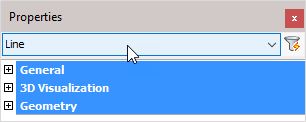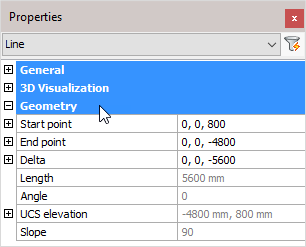The 'Line' object type has the following headings:

General

3D Visualization

GeometryGeneral: Is common to all object types, and covers general AutoCAD and BricsCAD settings, including: Color / Layer / Linetype / Linetype scale / Plot style / Lineweight / Transparency / Hyperlink / Handle /

3D Visualization: Is also common to all object types and includes: Material.

The material that is referred to here is only the visual representation material. For changing the actual material of parts, see the Additional Data section of plates, profiles or structures.

GeometryNote! - * indicates that the given value is a resultant of the sum of other values in the panel - and cannot be individually edited.

Start point: Line start point (X, Y, Z coordinates)

End point: Line end point (X, Y, Z coordinates)

Delta: Delta (X, Y, Z coordinates)

Length: * Calculated line length

Angle: * calculated line angle

UCS elevation: * UCS elevation (Reference)

Slope: * Slope angle relative to the horizontal plane (Reference)